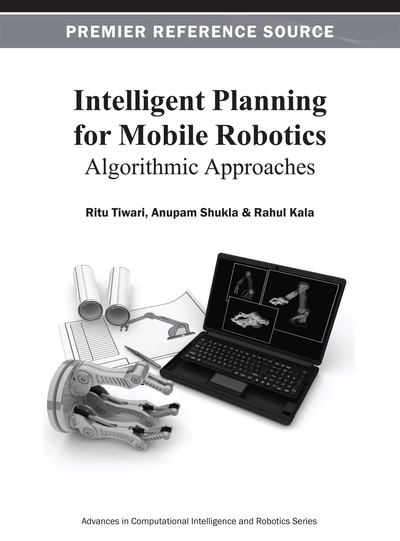# Graph Based Path Planning

DOI: 10.4018/978-1-4666-2074-2.ch002
Available
\$37.50
No Current Special Offers

## Abstract

Graphs are keenly studied by people of numerous domains as most of the applications we encounter in our daily lives can be easily given a graph-based representation. All the problems may then be easily studied as grap-based problems. In this chapter, the authors study the problem of robot motion planning as a graph search problem. The key steps involve the representation of the problem as a graph and solving the problem as a standard graph search problem. A number of graph search algorithms exist, each having its own advantages and disadvantages. In this chapter, the authors explain the concept, working methodology, and issues associated with some of these algorithms. The key algorithms under discussion include Breadth First Search, Depth First Search, A* Algorithm, Multi Neuron Heuristic Search, Dijkstra’s Algorithm, D* Algorithm, etc. Experimental results of some of these algorithms are also discussed. The chapter further presents the advantages and disadvantages of graph-based motion planning.
Chapter Preview
Top

## Introduction

Graphs have been of great interest to mathematicians for a long time. Graphs are able to model a large number of real life scenarios. The scenarios extend from game playing, where computers are used to play games like chess, to general everyday problems like the Hannibal and cannibals problem. The domains that the graphs touch are very wide. It is extremely exciting to see how the graphs model the various scenarios and further solve all the problems associated with them. Hence, graphs are constantly used by multiple people from multiple domains. A large number of graph algorithms have come up as well that do a variety of tasks and return the solutions. Graphs are commonly used to solve problems. These include social network analysis. Here, scientists used graph theory to analyze the social networks that are prevalent in all social networking sites. These may be further extended to research groups by figuring relations existing in citations or co-authors.

The discussion forums are further potential sources of networks that may be analyzed by using graph modeling techniques. Another major application of these algorithms is in the domain of planning and scheduling. Here, the scientists are interested in scheduling the various tasks in a project. This gives rise to automated project management and optimal resource utilization. Planning and scheduling may be easily converted into a graph by pointing relations and dependencies amongst the various sub-tasks. These may be then analyzed by graph algorithms for coming up with a strong plan of action regarding the project execution. The graphs have become useful tools for problem solving.

One of the classic benchmarks of graph search algorithms is the IBM Deep Blue project. Chess playing is seen as a challenging AI problem. Much like human players, the computer programs play chess intelligently by trying to forward compute all possible moves of them and the opponents, and try to figure out good moves. In 1997, IBM Deep Blue defeated Gary Kasparov, a world chess champion. Chess playing represents a highly complex graph with too many vertices and edges. It requires significant computing to search the graph in order to find out a move, which may be near optimal. This needs to be done within the timeframe as allowed in the game. The system used intensive parallel processing using high-end systems from IBM. A chess specific processor chip was developed by the team, which was proficient of evaluating and examining 2 to 3 thousand positions per second. It generated thirty billion positions for every move with an average depth of fourteen, routinely (IBM, 2011).

The numerous graph algorithms that are already extensively researched and designed play an important role in motivating conversion of all scenarios and problems into graphs. Hence, the entire task of problem solving gets divided into two parts. The first part, which is more application specific, is to concert the problem as a standard graph. This would require expertise and understanding of the problem. One further need to understand what exactly is the intended output of the system. Hence, the end deliverables must be precisely known. Once this step has been done, we may just be required to pick up a standard graph algorithm that can give us the desired output. The existence of a variety of standard graph problems and a variety of algorithms for each of these problems should usually mean that the algorithm for the task being desired is already available in the library.

Robot motion-planning is a problem that may be easily solved by using graphs. The problem here is twofold. The first part, or the major part deals with how we convert the motion-planning problem as a graph problem, deals with the representation aspects of the problem. The other half of the task is to simply use a standard graph search algorithm for working over the graph generated and to return the solution. We, hence, fundamentally divide the chapter into two parts. The first part deals with the problem of robot motion planning and its representation as a graph. The second part illustrates numerous graph search algorithms. The experimentation, where we apply some of these algorithms to get pictorial results, would be presented at the very end of the chapter. Before everything, it would be wise to brush up the concepts of graphs.

## Complete Chapter List

Search this Book:
Reset